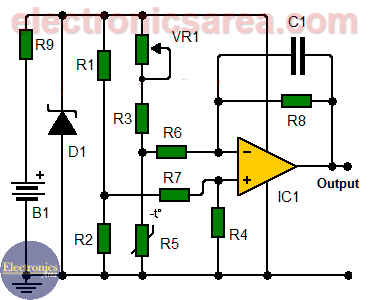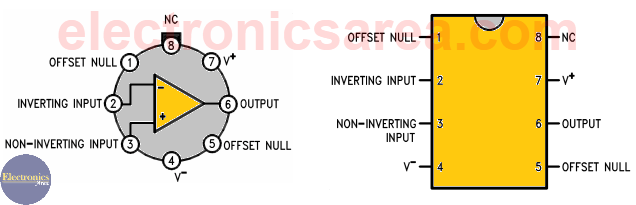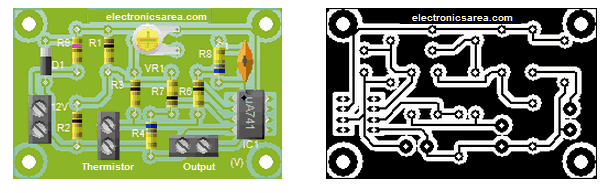# Temperature to voltage converter circuit using thermistor

This temperature to voltage converter circuit, convert the sensed temperature by a thermistor into a voltage level. The conversion can be considered linear within an acceptable temperature range. The nonlinearity of the conversion is due to characteristics of the thermistor. The circuit achieves an acceptable performance between 0°C and 24°C.

## How the Temperature to voltage converter works?

The change must be 0.5 volts per degree Celsius (°C). If this were not so, you must adjust the values of  the R4 and R8 resistors (the two resistors must have the same value). The temperature sensor (R5) is a negative coefficient thermistor. (NCT).

If temperature increases the resistive value of the thermistor decreases, as the voltage across its terminals. As a consequence the voltage, at the output terminal of the operational amplifier, increases.You can experiment with variations on the ranges of temperature, by changing the values of the R1 and R2 resistors (hint: put a 10K pot instead of R2)

## How To calibrate the temperature to voltage converter circuit?

To achieve an output of 0 volts, we decrease the thermistor temperature to 0°C, then we adjust the RV1 potentiometer to ensure that the output voltage is correct.## Temperature to voltage converter circuit list of components

• 1  741 operational amplifier (IC1)
• 3  10K resistors (R1, R2, R3)
• 2  680K resistors (R4, R8)
• 2  100K resistors (R6, R7)
• 1  470 ohm resistor (R9)
• 1  10K negative coefficient thermistor (NCT) (R5)
• 1  10K potentiometer (VR1)
• 1  1N4736, 6.8V zener diode (D1)
• 1  100 nF (nanoFarad) capacitor (C1)

## Printed Circuit Board (PCB)

A suggested printed circuit board and approximate appearance of the final circuit are shown.• 2
•
• 58
•
•
•
•
•
60
Shares
•
60
Shares
• 2
•
• 58
•
•
•
•
Tagged with: ,